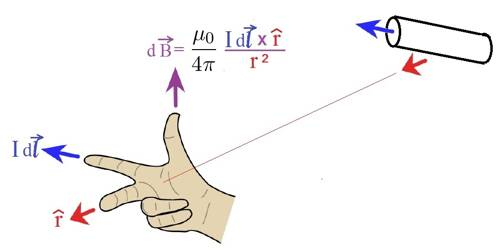Physics

# Biot-Savart’s Law or Laplace’s LawBiot-Savart’s Law or Laplace’s Law

Scientist Oersted discovered that there exists a magnetic field around a wire carrying current. To determine the magnetic induction or magnetic intensity of this magnetic field, scientist Laplace gave a theory or law. It is called Laplace’s theorem or

Later on, in 1820 scientists Biot and Savart gave an experimental proof of this law. Hence, it is called Biot-Savart’s Law. This law is applied to the generation of a magnetic field due to conductors of any shape and different current distributions. The law is stated below.

Law: The magnitude of magnetic field at a point produced around a conductor of small length due to the flow of current through it is-

(i) directly proportional to the amount of current.

(ii) directly proportional to the length of the conductor.

(iii) directly proportional to the sine of the angle between the lines connecting the point from the mid-point of the conductor and the conductor itself and

(iv) inversely proportional to the distance between the point and the mid-point of the conductor.

The Biot-Savart Law is an equation that describes the magnetic field created by a current-carrying wire and allows you to calculate its strength at various points.